##### How to Use AVG() Function in PostgreSQL

PostgreSQL provides a built-in AVG() function that is used to retrieve the average of a set. Postgres allows us to compute the average of distinct values using an additional option/operator, DISTINCT. While calculating the average of a set, the AVG() function skips the “NULL” values.

Let’s learn the working of the AVG() function through Practical examples.

How to Use AVG() Function in Postgres?

The below snippet demonstrates the basic syntax of the AVG() function:

`AVG(col_name);`

Let’s implement the AVG() function practically to get profound knowledge.

Example #1: How to Use AVG() Function on Table’s Data?

We have created a table named bike_details. Let’s execute the SELECT command to get all the records of the bike_details table:

`SELECT * FROM bike_details;`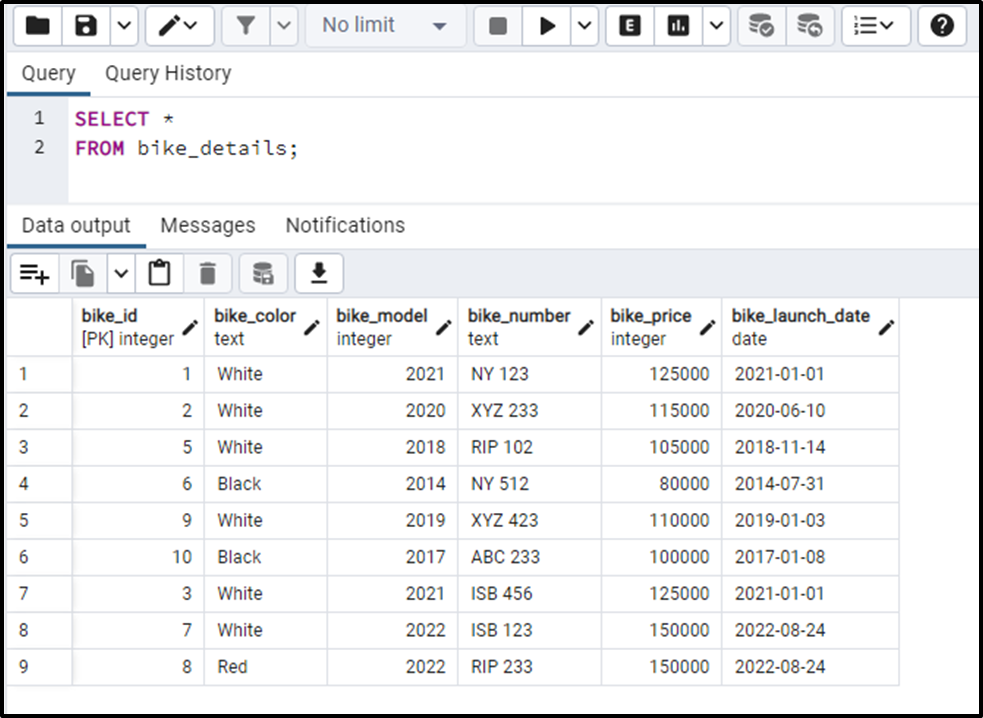Let’s compute the average of bike_price column using the AVG() Function:

```SELECT AVG(bike_price)
FROM bike_details;```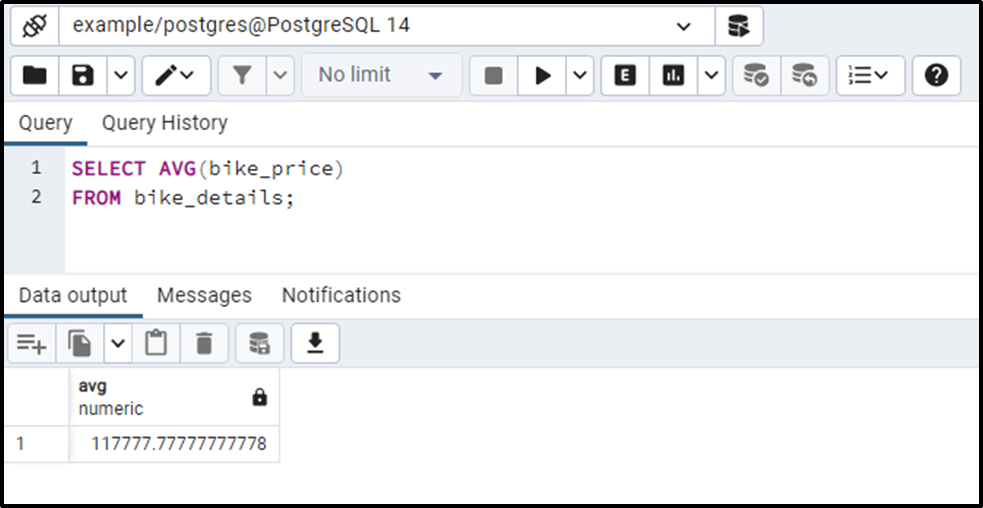Let’s run the below query to get the average up to specific decimal places:

```SELECT AVG(bike_price)::numeric(10, 3)
FROM bike_details;```

The above query will return the average of bike_price column in an easily understandable format, i.e., the AVG() will return the result up to three decimal places: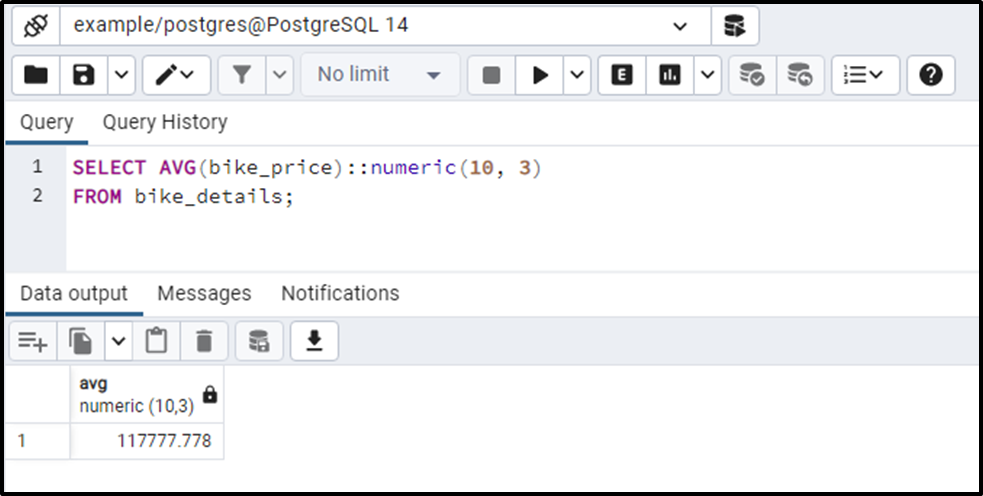Output proves the working of AVG() function.

Example #2: How Does the AVG() Function Deal With NULL Values in Postgres?

We inserted a new record to the bike_details table. Let’s check the updated table using the below command:

`SELECT * FROM bike_details;`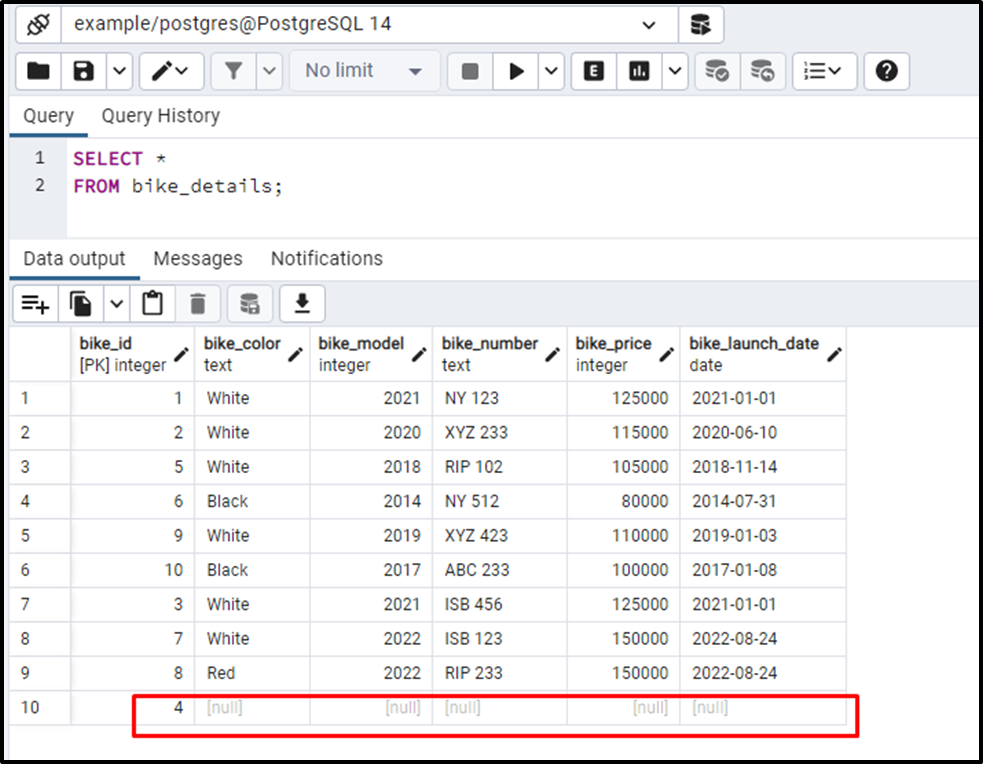We inserted a new record against the bike_id 4. Let’s run the below query to find the average of the bike_price column. The bike_details table contains some NULL values. This example will let you understand how the AVG() function deals with the NULL values in Postgres:

```SELECT AVG (bike_price):: NUMERIC(10, 2)
FROM bike_details;```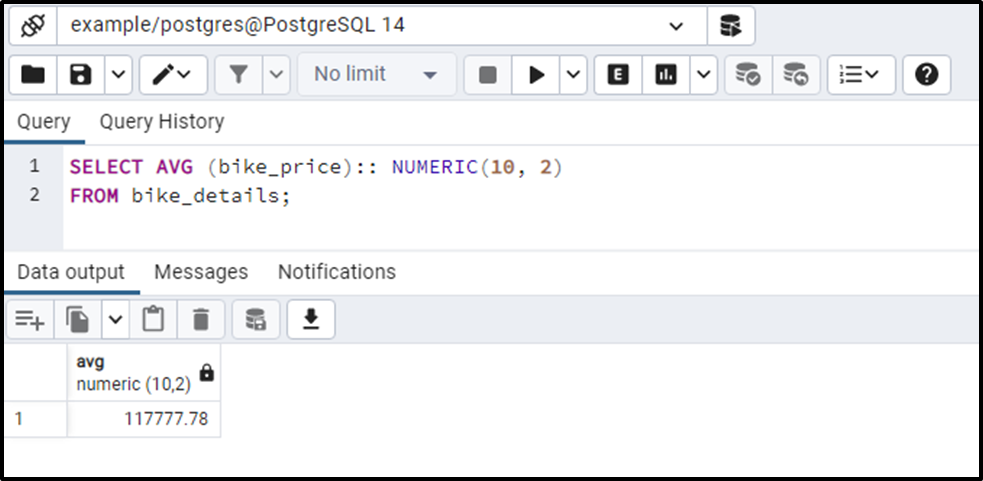The output shows that the AVG() ignores the NULL values.

Example #3: How to Use Distinct Operator With the AVG() Function?

Specify the DISTINCT operator before the column’s name to compute the average of the distinct values:

```SELECT AVG(DISTINCT bike_price):: numeric(10,2)
FROM bike_details;```

You will get the following results on successful execution of the above-given query: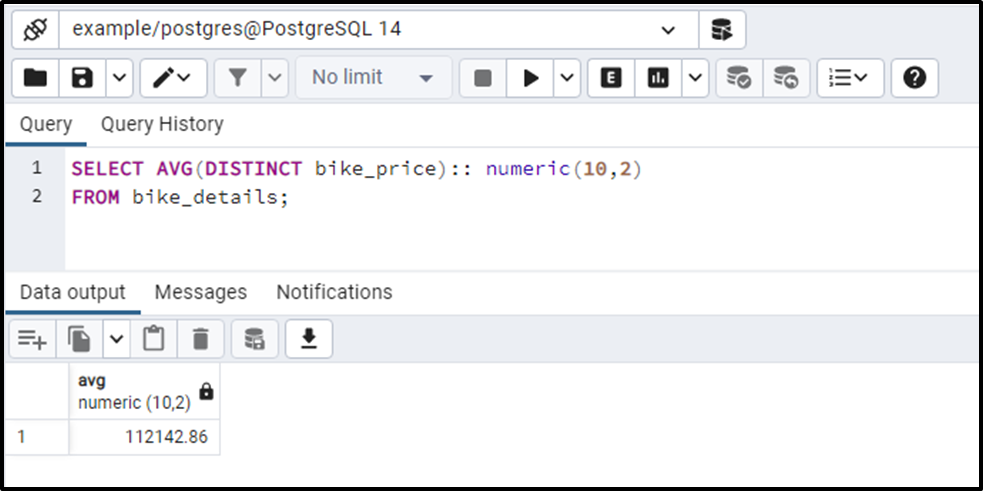The output proves that the AVG() function returns the average of distinct values.

Example #4: How to Use the AVG() Function to Compute Groups' Average?

Execute the below command to compute the average of the bike_price column based on the groups. To do that, utilize the GROUP BY clause as follows:

```SELECT
bike_model,
AVG (bike_price):: NUMERIC(10, 2)
FROM bike_details
GROUP BY bike_model;```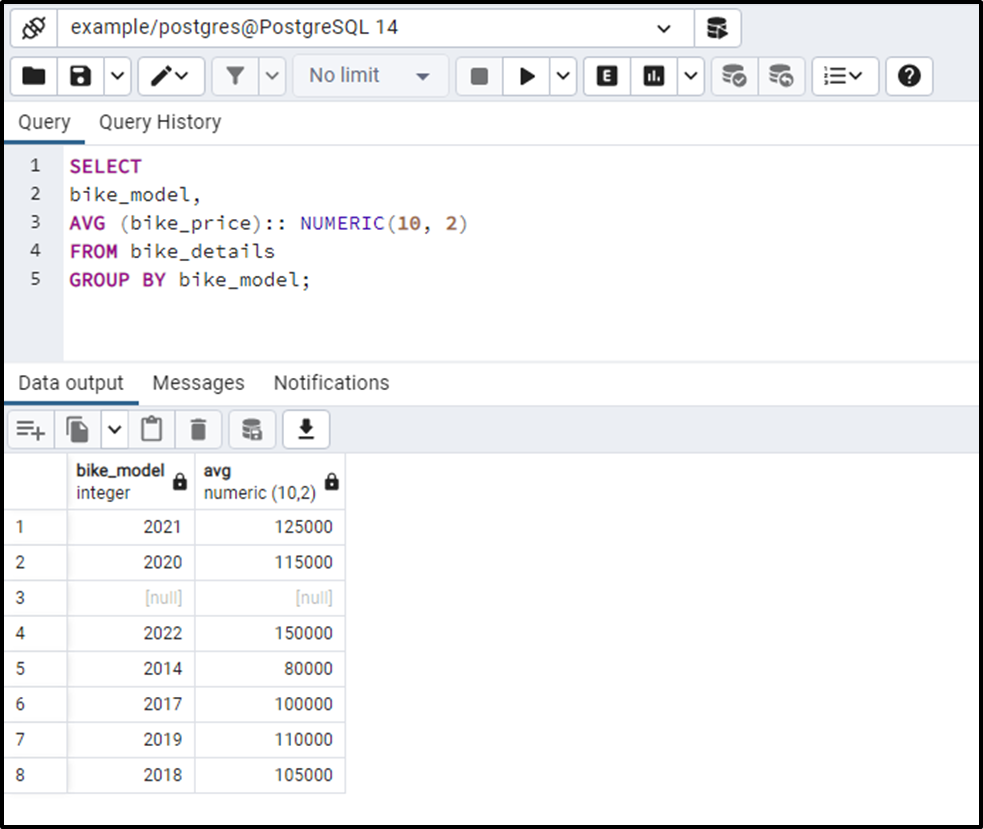From the output, you can observe that this time the AVG() function computed the average of groups.

Conclusion

PostgreSQL offers an in-built function named AVG() that returns the average of a set. Postgres allows us to compute the average of distinct values using an option/operator, DISTINCT. While calculating the average of a set, the AVG() function skips the “NULL” values. This write-up considered different scenarios to demonstrate the working of the AVG() function in PostgreSQL.